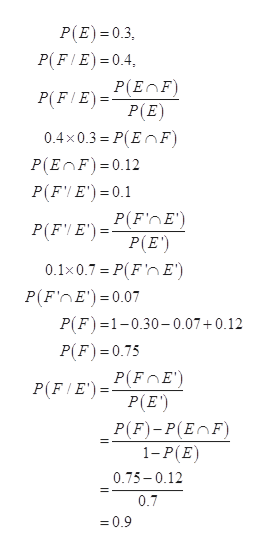# Let P(E)=0.3, P(F/E)=0.4, P(F'/E')=0.1. Finda) P(F/E')b) P(E intersects F)c) P(F') I couldnt find the intersect button so i wrote it out

Question
5 views

Let P(E)=0.3, P(F/E)=0.4, P(F'/E')=0.1. Find

a) P(F/E')

b) P(E intersects F)

c) P(F')

I couldnt find the intersect button so i wrote it out

check_circle

Step 1

Form the given information,

Step 2

Thus, the required probabilities ca...help_outlineImage TranscriptioncloseP(E) 0.3 P(F E) 0.4. Р(EnF) P(F E _ P(E) 0.4x0.3 P(EnF) P(EnF) 0.12 P(FE) 0.1 P(F'nE' P(F E')=P(E') 0.1x0.7 P(F'n E') P(F'nE')= 0.07 P(F)1-0.30-0.07+0.12 P(F) =0.75 P(FIE)PFOE') P(E') Р(F)-P(EnF) 1-P(E) 0.75-0.12 0.7 =0.9 fullscreen

### Want to see the full answer?

See Solution

#### Want to see this answer and more?

Solutions are written by subject experts who are available 24/7. Questions are typically answered within 1 hour.*

See Solution
*Response times may vary by subject and question.
Tagged in

### Basic Probability